# Leptons

Leptons and quarks are the basic building blocks of matter, i.e., they are seen as the "elementary particles". There are six leptons in the present structure, the electron, muon, and tau particles and their associated neutrinos. The different varieties of the elementary particles are commonly called "flavors", and the neutrinos here are considered to have distinctly different flavor.Important principles for all particle interactions are the conservation of lepton number and the conservation of baryon number.

Now that we have experimental evidence for six leptons, a relevant question is "Are there more?". The present standard model assumes that there are no more than three generations. One of the pieces of experimental evidence for that is the measured hydrogen/helium abundance ratio in the universe. When the process of nucleosynthesis from the big bang is modeled, the number of types of neutrinos affects the abundance of helium. The observed abundance agrees with three types of neutrinos.

 Table of lepton properties
Index

Particle concepts

 HyperPhysics***** Quantum Physics R Nave
Go Back

# Electron and Positron

As one of the leptons, the electron is viewed as one of the fundamental particles. It is a fermion of spin 1/2 and therefore constrained by the Pauli exclusion principle, a fact that has key implications for the building up of the periodic table of elements.

The electron's antiparticle, the positron, is identical in mass but has a positive charge. If an electron and a positron encounter each other, they will annihilate with the production of two gamma-rays. On the other hand, one of the mechanisms for the interaction of radiation with matter is the pair production of an electron-positron pair. Associated with the electron is the electron neutrino.

 Particle Symbol Anti-particle Rest massMeV/c2 L(e) L(muon) L(tau) Lifetime(seconds) Electron e- e+ 0.511 +1 0 0 Stable Neutrino(Electron)0(<7 x 10-6) +1 0 0 Stable
 Electron spin
 What evidence suggests that the electron is a fundamental particle?
Index

Particle concepts

 HyperPhysics***** Quantum Physics R Nave
Go Back

# Muon

The muon is a lepton which decays to form an electron or positron.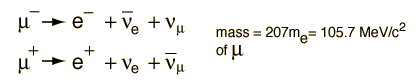The fact that the above decay is a three-particle decay is an example of the conservation of lepton number; there must be one electron neutrino and one muon neutrino or antineutrino in the decay.

The lifetime of the muon is 2.20 microseconds. The muon is produced in the upper atmosphere by the decay of pions produced by cosmic rays:Measuring the flux of muons of cosmic ray origin at different heights above the earth is an important time dilation experiment in relativity.

Muons make up more than half of the cosmic radiation at sea level, the remainder being mostly electrons, positrons and photons from cascade events. (Richtmyer) The average sea level muon flux is about 1 muon per square centimeter per minute.

 Particle Symbol Anti-particle Rest massMeV/c2 L(e) L(muon) L(tau) Lifetime(seconds) Muon μ- μ+ 105.7 0 +1 0 2.20x10-6 Neutrino(Muon)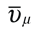0(<0.27) 0 +1 0 Stable
 Some history Atmospheric muons
Index

Particle concepts

 HyperPhysics***** Quantum Physics R Nave
Go Back

# Tau

The tau is the most massive of the leptons, having a rest mass some 3490 times the mass of the electron, also a lepton. Its mass is some 17 times that of the muon, the other massive lepton.

 Particle Symbol Anti-particle Rest massMeV/c2 L(e) L(muon) L(tau) Lifetime(seconds) Tau τ- τ+ 1777 0 0 +1 2.96x10-13 Neutrino(Tau)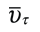0(<31) 0 0 +1 Stable

The tau lepton has spin 1/2 like the electron and muon leptons and an antiparticle with equal mass but opposite charge.

The tau lepton was detected in experiments at SLAC and LBL between 1974 and 1977. The discovery contributed to the Nobel Prize awarded to Martin Lewis Perl in 1995. The tau is the only lepton massive enough to decay into hadrons, particularly into pions.

Index

Particle concepts

Tau Wiki

 HyperPhysics***** Quantum Physics R Nave
Go Back

# Positron Annihilation

The positron is the antiparticle of the electron, and when a positron enters any normal matter, it will find an abundant supply of electrons with which to annihilate. The energy released by the annihilation forms two highly energetic gamma rays, and if one assumes that the momenta of the positron and electron were equal before the annihilation, the two gamma ray photons must travel in opposite directions in order to conserve momentum.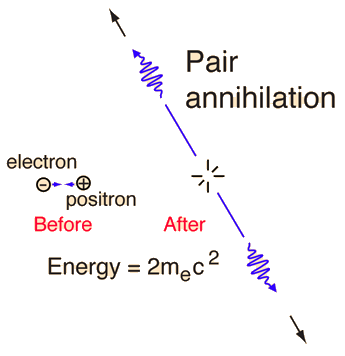These coincident gamma rays at 180 degrees provide a useful analysis tool. For one thing, eliminating all gamma events which are not coincident at 180 degrees improves the signal-to-noise ratio of experiments using positron annihilation. Another interesting application is the use of the coincident gammas to locate the source by back projecting. This is used in medical PET scans.
Index

Particle concepts

 HyperPhysics***** Quantum Physics R Nave
Go Back

# Electron-Positron Pair Production

When a photon has quantum energy higher than the rest mass energy of an electron plus a positron, one of the ways that such a photon interacts with matter is by producing and electron-positron pair.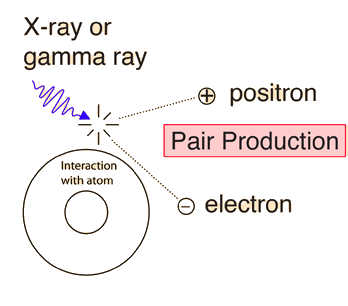The rest mass energy of the electron is 0.511 MeV, so for photon energy above 1.022MeV, pair production is possible. For photon energies far above this threshold, pair production becomes the dominant mode for the interaction of x-rays and gamma-rays with matter.

### More detail in relativity section

Index

Particle concepts

 HyperPhysics***** Quantum Physics R Nave
Go Back

# Properties of the Leptons

 Particle Symbol Anti-particle Rest massMeV/c2 L(e) L(muon) L(tau) Lifetime(seconds) Electron e- e+ 0.511 +1 0 0 Stable Neutrino(Electron)0(<7 x 10-6) +1 0 0 Stable Muon μ- μ+ 105.7 0 +1 0 2.20x10-6 Neutrino(Muon)0(<0.27) 0 +1 0 Stable Tau τ- τ+ 1777 0 0 +1 2.96x10-13 Neutrino(Tau)0(<31) 0 0 +1 Stable
Numerical data from Giancoli

 Lepton discussion
Index

Particle concepts

Reference
Giancoli

 HyperPhysics***** Quantum Physics R Nave
Go Back